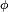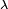# Geographic coordinate system facts for kids

Kids Encyclopedia Facts
(Redirected from Geographic coordinates)

A geographical coordinate system is a coordinate system. This means that every place can be specified by a set of three numbers, called coordinates.

A full circle can be divided into 360 degrees (or 360°); this was first done by the Babylonians; Ancient Greeks, like Ptolemy later extended the theory.

Today, degrees are divided further. There are minutes, and seconds; 1 minute (or 1') in this context is 1/60 of a degree; 1 second (or 1") is 1/60 of a minute.

The first concept needed is called latitude (Lat, or the Greek symbol "phi",$\scriptstyle{\phi}\,\!$). For it, the Earth is cut up into 180 circles, from the Equator at 0°. The poles are at 90°, the North Pole is at 90° N(orth), the South Pole is at 90° S(outh). Places with the same latitude are on a circle, around the Earth.

The other concept is called longitude (Long, or the Greek symbol "lambda",$\scriptstyle{\lambda}\,\!$), sometimes referred to as "meridian". The 0° longitude line (or zero meridian) goes through the Royal Observatory in Greenwich. Greenwich is a part of London. Then lines are drawn in a similar way; the opposite (or "antipodal") meridian of Greenwich is considered both 180°W(est), and 180°E(ast).

The third number is the height, altitude, or depth. This is given with respect to some fixed (usually easily calculable point). One of these is called mean sea level.Geographic coordinate system Facts for Kids. Kiddle Encyclopedia.